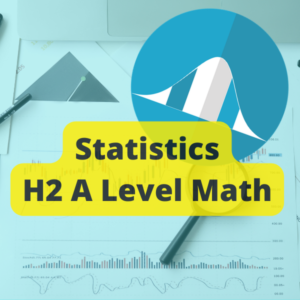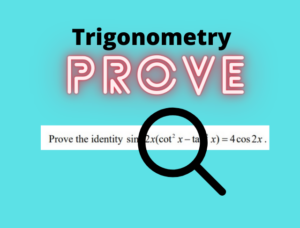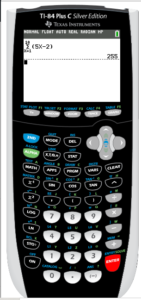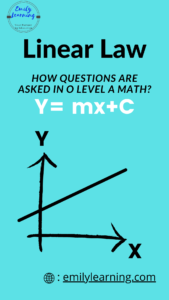# Category: Math

Here, you will find resources on O Level and A Level Mathematics resources.## Permutation and Combination Questions: A Level TYS Answers

Find the worked solutions for past A Level permutation and combination questions. Permutation and combination questions are found in paper 2.## Use of Ti 84 for normal distribution

We talk about the graphic calculator Ti-84 for normal distribution in this post. We’ll look at normal distribution functions in Ti-84: invNorm and normcdf.## Use of Ti 84 for binomial distribution

We talk about the graphic calculator Ti 84 for binomial distribution in this post. We’ll look at 2 binomial distribution functions in Ti 84.## A Level Math Statistics Questions – Past Year Papers by Topics

In this post, you will find the 2017 to 2022 H2 A Level Math statistics questions arranged by topics. Click on the topic to see the questions.## H2 A Level Math Statistics – Summary of Formulae

Find a summary of A Level Math Statistics in this post here. We go through key concepts on all topics tested in H2 A Level Math Statistics.## Proving Trigonometry Identity for O Level Additional Mathematics

Proving Trigonometry Identity Proving trigonometry identity is a common type of questions often tested In O Level Additional Mathematics. To prove an identity, students are…## Using Graphic Calculator for AP GP Questions ( Arithmetic and Geometric Progression)

In this post, let’s look at the use of graphic calculator for AP GP questions. You need the graphic calculator to solve some types of…## Graphic Calculator for A Level H2 Math

Having a graphic calculator for A Level H2 Math exam is a must for students. There aren’t many types of graphic calculators approved for the…## Linear Law A Math Questions are Predictable

Linear Law questions in A Math are very predictable! Because questions from this topic are predictable, it is easy to score for Linear Law O…## Suggested Solutions: 2019 GCE O Level A Math Paper 1

Here, you will find the suggested answer for 2019 GCE O Level A Math exam Paper 2. Click on the link below to go to…